## Applications of Gauss’s Law

1. Field due to an infinitely long straight uniformly charged wire
2. Field due to uniformly charged infinite plane sheet
3. Field due to a uniformly charged thin spherical shell

### Field due to an infinitely long straight uniformly charged wire

Consider an infinitely long thin straight wire with uniform linear charge density λ. The direction of electric field at every point must be radial (outward if λ > 0, inward if λ < 0).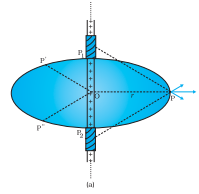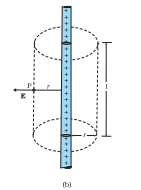Consider a pair of line elements P1 and P2 of the wire, as shown. The electric fields produced by the two elements of the pair when summed give a resultant electric field which is radial (the components normal to the radial vector cancel).

To calculate the field, imagine a cylindrical Gaussian surface, as shown in the Fig. Since the field is everywhere radial, flux through the two ends of the cylindrical Gaussian surface is zero.

At the cylindrical part of the surface, E is normal to the surface at every point, and its magnitude is constant since it depends only on r.

The surface area of the curved part is 2πrl, where l is the length of the cylinder.

Flux through the Gaussian surface = Flux through the curved cylindrical part of the surface

= E × 2πrl

The surface includes charge equal to λ l. Gauss’s law then gives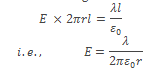Vectorially, E at any point is given by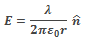where nˆ is the radial unit vector in the plane normal to the wire passing through the point. E is directed outward if λ is positive and inward if λ is negative.

### Field due to uniformly charged infinite plane sheet

Let σ be the uniform surface charge density of an infinite plane sheet. We take the x-axis normal to the given plane. By symmetry, the electric field will not depend on y and z coordinates and its direction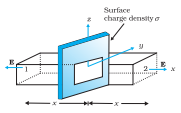We can take the Gaussian surface to be a rectangular parallelepiped of cross-sectional area A, as shown. (A cylindrical surface will also do.) As seen from the figure, only the two faces 1 and 2 will contribute to the flux; electric field lines are parallel to the other faces and they, therefore, do not contribute to the total flux.

The unit vector normal to surface 1 is in –x-direction while the unit vector normal to surface 2 is in the +x direction. Therefore, fluxes E.ΔS through both the surfaces are equal and add up. Therefore the net flux through the Gaussian surface is 2 EA. The charge enclosed by the closed surface is σ A. Therefore by Gauss’s law,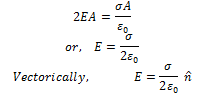where nˆ is a unit vector normal to the plane and going away from it. E is directed away from the plate if σ is positive and toward the plate if σ is negative.

### Field due to a uniformly charged thin spherical shell

Let σ be the uniform surface charge density of a thin spherical shell of radius R. The field at any point P, outside or inside, can depend only on r (the radial distance from the center of the shell to the point) and must be radial (i.e., along with the radius vector).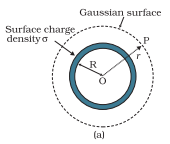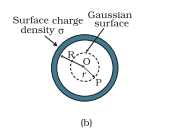#### The field outside the shell:

Consider a point P outside the shell with radius vector r. To calculate E at P, we take the Gaussian surface to be a sphere of radius r and with center O, passing through P. All points on this sphere are equivalent relative to the given charged configuration. The electric field at each point of the Gaussian surface, therefore, has the same magnitude E and is along the radius vector at each point.

Thus, E and ΔS at every point are parallel and the flux through each element is E ΔS. Summing over all ΔS, the flux through the Gaussian surface is E × 4 π r2. The charge enclosed is σ × 4 π R2. By Gauss’s lawWhere q = 4πR2σ is the total charge on the spherical shell.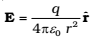The electric field is directed outward if q > 0 and inward if q < 0. This, however, is exactly the field produced by a charge q placed at the center O. Thus for points outside the shell, the field due to a uniformly charged shell is as if the entire charge of the shell is concentrated at its center.

#### The field inside the shell:

In Fig., point P is inside the shell. The Gaussian surface is again a sphere through P centered at O.

The flux through the Gaussian surface, calculated as before, is E × 4 π r2. However, in this case, the Gaussian surface encloses no charge. Gauss’s law then gives E × 4 π r2 = 0 i.e., E = 0 (r < R).

The field due to a uniformly charged thin shell is zero at all points inside the shell. This important result is a direct consequence of Gauss’s law which follows from Coulomb’s law.